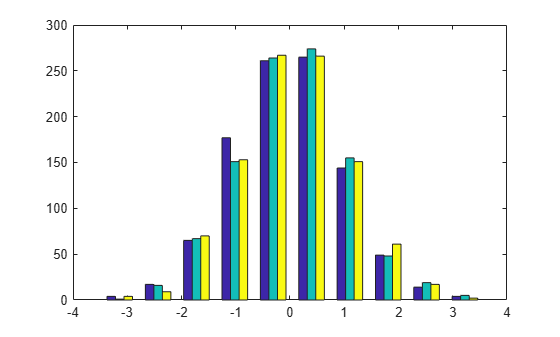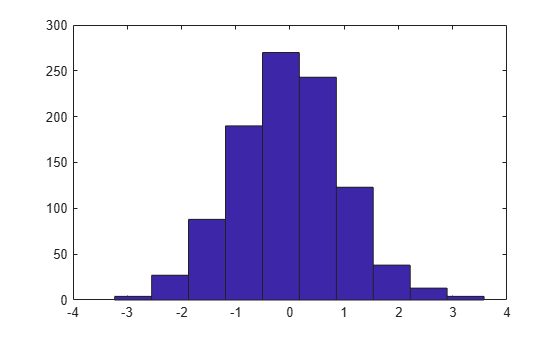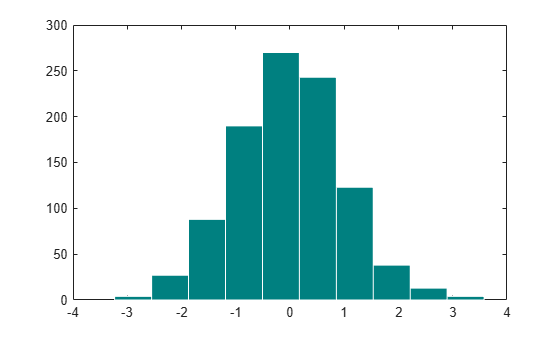# hist

Histogram plot (not recommended; use `histogram`)

`hist` is not recommended. Use `histogram` instead.

For more information, including suggestions on updating code, see Replace Discouraged Instances of hist and histc.

## Syntax

``hist(x)``
``hist(x,nbins)``
``hist(x,xbins)``
``hist(ax,___)``
``counts = hist(___)``
``````[counts,centers] = hist(___)``````

## Description

example

````hist(x)` creates a histogram bar chart of the elements in vector `x`. The elements in `x` are sorted into 10 equally spaced bins along the x-axis between the minimum and maximum values of `x`. `hist` displays bins as rectangles, such that the height of each rectangle indicates the number of elements in the bin.If the input is a multi-column array, `hist` creates histograms for each column of `x` and overlays them onto a single plot.If the input is of data type `categorical`, each bin is a category of `x`.```

example

````hist(x,nbins)` sorts `x` into the number of bins specified by the scalar `nbins`.```
````hist(x,xbins)` sorts `x` into bins with intervals or categories determined by the vector `xbins`. If `xbins` is a vector of evenly spaced values, then `hist` uses the values as the bin centers.If `xbins` is a vector of unevenly spaced values, then `hist` uses the midpoints between consecutive values as the bin edges.If `x` is of data type `categorical`, then `xbins` must be a categorical vector or cell array of character vectors that specifies categories. `hist` plots bars only for those categories. The length of the vector `xbins` is equal to the number of bins.```
````hist(ax,___)` plots into the axes specified by `ax` instead of into the current axes (`gca`). The option `ax` can precede any of the input argument combinations in the previous syntaxes.```
````counts = hist(___)` returns a row vector, `counts`, containing the number of elements in each bin.```

example

``````[counts,centers] = hist(___)``` returns an additional row vector, `centers`, indicating the location of each bin center on the x-axis.```

## Examples

collapse all

```x = [0 2 9 2 5 8 7 3 1 9 4 3 5 8 10 0 1 2 9 5 10]; hist(x)````hist` sorts the values in `x` among 10 equally spaced bins between the minimum and maximum values in the vector, which are 0 and 10 in this example.

Generate three columns of 1,000 random numbers and plot the three column overlaid histogram.

```x = randn(1000,3); hist(x)```The values in `x` are sorted among 10 equally spaced bins between the minimum and maximum values. `hist` sorts and bins the columns of `x` separately and plots each column with a different color.

Plot a histogram of 1,000 random numbers sorted into 50 equally spaced bins.

```x = randn(1000,1); nbins = 50; hist(x,nbins)```Generate 1,000 random numbers. Count how many numbers are in each of 10 equally spaced bins. Return the bin counts and bin centers.

```x = randn(1000,1); [counts,centers] = hist(x)```
```counts = 1×10 4 27 88 190 270 243 123 38 13 4 ```
```centers = 1×10 -2.8915 -2.2105 -1.5294 -0.8484 -0.1673 0.5137 1.1947 1.8758 2.5568 3.2379 ```

Use `bar` to plot the histogram.

`bar(centers,counts)`Generate 1,000 random numbers and create a histogram.

```data = randn(1000,1); hist(data)```Get the handle to the patch object that creates the histogram plot.

`h = findobj(gca,'Type','patch');`

Set the face color of the bars plotted to an RGB triplet value of `[0 0.5 0.5]`. Set the edge color to white.

```h.FaceColor = [0 0.5 0.5]; h.EdgeColor = 'w';```## Input Arguments

collapse all

Input vector or matrix.

• If `x` is a vector, then `hist` creates one histogram.

• If `x` is a matrix, then `hist` creates a separate histogram for each column and plots the histograms using different colors.

If the input array contains `NaN`s or undefined categorical values, `hist` does not include these values in the bin counts.

If the input array contains the infinite values `-Inf` or `Inf`, then `hist` sorts `-Inf` into the first bin and `Inf` into the last bin. If you do not specify the bin intervals, then `hist` calculates the bin intervals using only the finite values in the input array.

Data Types: `single|double|logical|categorical`

Number of bins. Input `x` must be numeric, not categorical.

Data Types: `single|double|int8|int16|int32|int64|uint8|uint16|uint32|uint64`

Bin locations or categories, specified as a vector.

If `x` is numeric or logical, then `xbins` must be of type `single` or `double`.

• If the elements in `xbins` are equally spaced, then these elements are the bin centers.

• If the elements in `xbins` are not equally spaced, then these elements are indicated by markers along the x-axis, but are not the actual bin centers. Instead, `hist` calculates the bin edges as the midpoints between consecutive elements in vector `xbins`. To specify the bin edges directly, use `histc`.

• `xbins` must contain only finite values. The first and last bins extend to cover the minimum and maximum values in `x`.

If `x` is categorical, then `xbins` must be a categorical vector or cell array of character vectors that specifies categories. `hist` plots bars only for those categories specified by `xbins`.

Axes object. Use `ax` to plot the histogram in a specific axes instead of the current axes (`gca`).

## Output Arguments

collapse all

Counts of the number of elements in each bin, returned as a row vector.

Bin centers or categories, returned as a vector. If used with the syntax `[counts,centers] = hist(x,xbins)`, then the `centers` output has the same elements as the `xbins` input.

• If `x` is numeric or logical, then `centers` is a numeric row vector.

• If `x` is categorical, then `centers` is a cell array of character vectors.

## Version History

Introduced before R2006a